# Better Math Solutions (than what the College Board will give you)

The College Board would like you to believe that they are on your side. To help convince you of this, they have provided many resources to help you prepare for the SAT. One essential resource: actual practice SATS. You can get them here. Taking real practice tests is certainly the key element in an effective prep plan. So it is nice of the College Board to provide you with them. And they even give you answers and solutions. How kind of them!

But one way to tell that they are not sincerely trying to helping you is by examining those math solutions. Yes, the solutions are scrupulously correct. A bit dry maybe. Sometimes stark and intimidating, but nevertheless, quite correct.

But there is an omission so glaring, so disingenuous, that the only explanation is that they want to claim the appearance of being helpful while still maintaining their own agenda. (World domination? Eventually. But for now, they would like to be the official end-of-high-school test in every state.)

If they were honest, the solutions would open with something like this:

Every SAT problem can be solved by a formal application of the mathematical concepts and procedures you have been learning in high school. We believe that this knowledge is important for success in many fields of study in college. So we are presenting solutions that exercise those skills in that formal way you have seen. If you learn to solve these problems in this particular way, your score on the SAT will certainly improve.

I believe the College Board would not object to that last paragraph. But here is what I wish they would say next:

But we also recognize that many of the problems on the SAT can be solved in alternative ways, using less formal (but more creative) mathematical approaches. We present those solutions here as well. Learning to solve problems in this manner will also help your score to improve. While these methods occasionally seem to circumvent some of the required math knowledge, they also promote a flexibility and creativity that will serve you well in your future studies.

I am emphatically not “anti-math” in any way. I like algebra! But if we want to help people to improve their SAT scores, we should not be holding back this information. And if you happen to be a little shaky in the formal methods of algebra, that is hard to fix in a shorter (say a few months) time frame. Learning another way to play can really open some doors for you. There is no way that the College Board does not know about these methods. But you see no evidence of that in their solutions. I guess they want to help you improve but only if you are willing to do it their way.

To illustrate what I am talking about, I am going to provide a few examples from the most recent practice test, #10. (I have a longer set of these for tests 1 – 8 here and I will try to update the collection soon.)

I hope that is a large enough sample to convince you that these approaches are potentially useful. I have seen them work a substantial number of times on every SAT that has ever been released. Also, it is good to have more than one way to approach a question. That gives you a way to stay in the game even when you don’t feel you know the “right” way to do a problem.

From Test #10, Section 3:

Again, I am not saying that these are the fastest solutions. Please don’t feel that you have to email or tweet at me with your algebra. These are ALTERNATIVE solutions! (And not every question has one…that I have found yet!)

1.You could try each answer, plugging in until you find one that works.

2. Ignore the algebra and just play around until you find how many weeks it will take: You after the initial \$60 payment, you still owe \$240. At \$30 per week, it will take 8 weeks. Aha! Now plug in w=8 into each answer choice…

3. From the table, we can get a sample value. For example, when x = 10, the answer is supposed to come out to \$21.89 . So try x = 10 in each answer choice. If you don’t get \$21.89, it’s wrong.

5. Make up a number for x…like x=4. You get the square root of 144 which is 12. Now put x=4 into each answer choice….

6. Seriously, carefully trying each answer is quicker and easier than doing the algebra…

9. Go to the graph and find a point that is on the line. I used (-2,-2). That means that we can put x=-2 and y=-2 into the equation and it should work. Only one does! (If more than one worked, I would try another point.)

11. Draw a neat diagram of a circle centered at (5,7) with a radius of 2. Find any point on that circle. For example, 2 units to the right is (7,7). Then, see which equation works when you use x=7, y=7…

14. Again, trying their answers is a lot easier than doing the algebra. But this question is obnoxious. It targets the students who do not know that the square root symbol means the POSITIVE number that squares to give you what you want.

20. I cannot believe that they did not mention this solution: you could solve for u and t. That seems to require algebra, but you can also do it by playing with numbers. We need numbers whose sum is 5 and difference is 2. Let’s see: 4+1=5 but 4-1=3…too big. 2+2=4 but 2-2=0…too small. Uh oh. Fractions? 3.5 + 1.5 = 5. 3.5-1.5 = 2. Nice. You still have to do the arithmetic though…

OK that’s all for now. I will eventually post more of these for Section 4 and for Test #9. And I am pondering a way to turn this into a group project. I know a good number of fellow SAT nerds. So here is a heads-up to them: I may be reaching out soon to see if you want to help build a collection of these in some free, publicly available format. Stay tuned…

# Lessons from Math SAT Prep for the Math Classroom

A brief rant

In light of the on-going controversy regarding admission to New York City’s selective high schools, there has been some discussion of bringing test prep to the middle school classroom.  At first, test prep in the math classroom may sound like an awful idea.  Should we take what is frequently, sad to say, a joyless, stultifying slog and add in a large helping of stress-inducing rote drill to prepare for the SHSAT, PSAT, SAT, what have you?  That can’t be a good idea. And yet…

Maybe we should work on fixing the joyless, stultifying part.  I have taught (oh, lord) a ton of SAT prep.  None of it involves rote drills.  It does involve a number of ideas and methods that absolutely help make math more joyful.  And many, many students have come into my SAT class announcing that they don’t like math only to later report that they “kind of like” SAT math.   So what is happening in SAT class that isn’t happening often enough in school?  Here are some possible answers:

1. SAT math is subversive.  And being subversive is fun.  You don’t have to solve the problem the “right” way!  You can play, guess, draw pictures, make lists…and if it works, you get full credit!  School math is often presented like some deranged cooking class where everyone learns to cook the same dish in the exact same way. No invention, no experimentation, no surprises. (And it’s rarely anything anyone wants to eat anyway.)  We should be encouraging mathematical improvisation and creativity – even when it doesn’t “work”.  After all, how often do we really care where the vertex of the parabola is?  Isn’t the more valuable experience the search?  A math problem that only has one solution path isn’t really a “problem” — it’s more like a “chore”.  No one likes doing chores.

2. SAT math rewards trial and error.  School math often treats trial and error as a tool for the weak.  But trial and error is a fabulous way to begin a problem when you don’t see an algebraic path.  It is not merely an algebra-evasion technique (though on the SAT, it can certainly work that way). Trial and error can serve as an on-ramp to the algebraic solution.  I have used trial and error this way with students in SAT class, physics, precalc, calculus, and multivariable calc.  To keep this rant brief (well…) I will expand on and demonstrate this idea in a separate post.  But to give you the flavor:

1. You take a guess (or pick an answer if it is a multiple choice test)

2. You mess around with your guess, applying whatever line of reasoning you can come up with to show that your guess is wrong (as it usually will be).

3. You replace your guess with a variable and then use your earlier reasoning as a template to come up with the algebraic equation or equations that will lead to an answer.

I have shown this method to students as young as 6th grade and to colleagues who have been teaching for decades.  I can tell you that most of them got a kick out of this.  Again, it feels subversive. And who doesn’t like the feeling that you are getting away with something?  But really, you’re not. You are just separating the problem into two phases, doing the reasoning before you attempt to apply the algebra.  But it only works if you are willing to take a guess. “Could this be the answer?” is an amazingly helpful question, and not just on a standardized test.

3. SAT math rewards making up numbers for variables.  This one is an SAT classic that should really be a staple of school math as well.  Many students see an algebraic equation as a sequence of letters and symbols, probably including an “=” somewhere in there.  We want them to see it as a statement, a sentence written in the language of algebra, one that might be true if the variables have the “right” values.

This gives us an efficient way of finding whether two expressions are equivalent.  Make up numbers (ideally, slightly “unusual” ones), put them into both expressions, and see if they come out equal.  And yes, this method can generate false positives. But it never generates false negatives – if you get two different numeric answers, then your two algebraic expressions were definitely not equivalent.

This idea has many uses.  First of all, we can teach students that arithmetic is a testing bench for algebra.  Any time you are not certain whether something is allowed, try it with numbers!  We all have taught plenty of students who believe that (x + y)2 = x2 + y2.  So let x =4 and y = 6 and see what happens!

Secondly, it inoculates students from the classic standardized test question: “which of the following expressions is equivalent to…” , a type of question that deserves to be subverted.

And thirdly, once again it gives us a way to separate the reasoning from the algebra.  You can make up numbers for the variables, work out an answer, and then put your variables back into the answer choices.  It’s probably the SAT method that seems most devious. Even in physics class, often my beginning students are flummoxed by questions like:

A car has mass m and speed v.  A second car has half the mass and three times the speed. Find the ratio of the second car’s kinetic energy to the first car’s kinetic energy.

The problem is not that these students don’t know the formula for kinetic energy. It’s that they have not yet developed the ability to think about the ratios and proportions involved.  When they are fluent, they will be able to look at the formula, K = ½ mv2 and think through the problem with ease.  But while they are still developing, isn’t it nice to have an alternative scaffold available?

Make up numbers for m and v and calculate the kinetic energy.

Cut the m in half  and triple the v and recalculate the kinetic energy.

Beginners are often surprised that this works, and even skeptical, thinking that there was something special about the numbers they made up. So let them make up a new set of numbers.    It seems like magic. And again, it serves as an on-ramp to the algebraic discussion.

Two more points and I’ll call it a rant

1. None of what I am recommending will take time away from your curriculum. These are attitudes and strategies that can overlay whatever you are currently teaching. Still, take a hard look: it isn’t all joyful now and it isn’t all as essential as you may think.  But we can argue about that another time.  I would teach these methods even if the SAT and its ilk were to vanish.  It’s a more fun way to do math.

2. Test prep in middle school is not going to magically bring diversity to the elite high schools. It might help, but I would guess that it won’t be nearly enough.  We are going to have to do more.

# Logarithms as Digit-Counters in ANY base!

File this under “math I should have realized”.

Even after all these years, I still find logarithms to be challenging, compelling, and surprising.  This post is about an idea that snuck up on me this morning. And it snuck up even though:

• I have written: Log2 8 = 3 because 23 = 8 on many whiteboards and many review sheets.
• I have learned (and taught!) the classic collection of assorted log properties
• I have read David Mermin’s lovely piece “Logarithms!” (and blogged about it here.)
• I have written a chapter on exponential functions and logarithms in “Advanced Math for Young Students”.

And yet, there is a basic idea about logarithms that I never really thought about (until this morning).  So here it is:

The integer part of the logarithm of an integer is one less than the number of digits the integer has when it is represented in the same base as the logarithm itself.

You could write:

Floor[Logb  N] + 1 = the number of N’s digits when N is represented in base b.

In other words, the logarithm counts the digits — and not just in base-10. You just have to round down and add 1.  I would like to explain this, if for no other reason than to convince myself that it is true!

Starting with base 10:

Let’s look at some common (base 10) logarithms that we can find without a calculator:

Log 1 = 0               …because 100 = 1

Log 10 = 1            …because 101 = 10

Log 100 = 2          …because 102 = 100

Log 1000 = 3        …because 103 = 1000

Log 10000 = 4     …because 104 = 10000

I think you get the idea.  If we construct a number, say N, by writing a 1 followed by k zeroes, then the common (base 10) logarithm, log N = k.  And the number will have k + 1 digits: the k zeroes and the 1 in front of them. So for each of these, adding 1 to the common logarithm gives you the number of digits.

Now let’s look at one that you do need a calculator for. I’ll report three decimal places:

Log 375 = 2.574   …because 102.574 = 374.973 ≈ 375

And it makes sense: 375 is more than 100 so its common logarithm is greater than 2.  But 375 is also less than 1000 so its common logarithm is less than 3.  It is more than the smallest 3 digit number and less than the smallest 4 digit number.    And if you take the common logarithm, which was 2.574, round it down and then add 1, you get the number of digits.  Nice.

Let’s try another (again reporting 3 decimal places):

Log (8787) = 3.944   … because 103.944  ≈ 8787 (though with a little more round-off error, but still…)

And this makes sense too: 8787 is more than 1000 so its common log is greater than 3.  But it is less than 10000 so its common log is less than 4.  In fact, it is closer to 10000 so its log is close to 4, but still less.  And if we round down 3.944 and add 1, again we get the number of digits in the number we started with.

OK, I admit that I kind-of knew that already.  But for some reason, this next step caught me by surprise…let’s move away from base 10 and see what happens.

Other bases and other logarithms

On and off this year, I have been playing with James Tanton’s invention, “Exploding Dots”.  If you have not yet seen this, you really should take a look.  You can get started here. But really, Google it – those dots are not just exploding, they are proliferating!

One thing that Exploding Dots teaches you almost immediately and organically is how to express numbers in other bases.  And something interesting happens when you look at the base 2 logarithms as you look at the base 2 representations…

So if a number N happens to be an integer power of 2, say 2k, then we know two things:

1. Its base-2 logarithm, log2 N = k

2. Its base-2 representation will consist of k zeroes and a leading 1.  And that makes k + 1 digits.

Now let’s get some help from a calculator.

Log2 5 = 2.322 …because 22.322  ≈ 5

Does it make sense? Well, since 5 is more than 4, we expect its base-2 logarithm to be more than 2.  But 5 is less than 8, so we expect its base-2 logarithm to be less than 3.  So this looks right.

But we are not done! If we explode some dots, we can find the base 2 representation.

5 = 1 0 1 and that makes 3 digits – which is also what we get when we round down the base-2 logarithm and then add 1!

OK, let’s check a big number:

500 expressed in base 2 is 1 1 1 1 0 1 0 0 (which you might want to check…)

I see 9 digits.

Log2 500 = 8.966 (and yes, it checks out: 28.966  ≈ 500)

Round it down and add 1…YES!

So if you want to know the number of digits in the base-b representation of a number, find the base-b logarithm, round it down to its floor and then add one.  The logarithm is the digit counter!

# A Magical Theorem, Part II: Areas by way of Antiderivatives

So how are areas related to antiderivatives?  In other words (and symbols):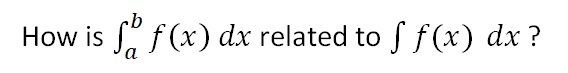This is the fundamental question.  When you know the answer, you will know the “Fundamental Theorem of Integral Calculus”.

In your first physics class, while studying kinematics, you learned that the area under a velocity vs time graph tells us the change in the object’s position.  To help jog your memory, play the video below:

The upper graph shows the velocity as a function of time for an object that happened to have a constant velocity of 10 m/s.

The lower graph shows the position vs time for the same object.  The velocity is constant so the position graph is linear with a constant slope.

On the velocity graph, you can see the area being swept out from t = 3 s to t = 7 s.  And on the position graph, you can see how far the object has moved during the same time interval.  Try stopping the animation at different times. Examine the graphs until you have convinced yourself of the following claim:

For a given time interval, the area under the Velocity vs. time graph will be equal to the change in position during that time interval.

YOU MAY BE SKEPTICAL…

After all, that was just with constant velocity.  But what if the velocity is changing?  Like so…

The velocity is increasing linearly and the position is increasing quadratically. [Remember, velocity is the derivative of position with respect to time. And the derivative of a 2nd-degree polynomial is a linear function.]

But even so, once again we see:

For a given time interval, the area under the Velocity vs. time graph will be equal to the change in position during that time interval.

STILL SKEPTICAL? STAY TUNED…

The velocity does not have to be changing linearly.  In fact, it does not even have to be velocity and position that we are talking about.  It can be any two quantities where one is the derivative of the other. Here’s one where the first graph is the rate at which water flows into a container and the second graph is the volume of water in that container:

Stop the animation at various points to check our claim:

If f(x) is the derivative of F(x), then the area under the graph of f(x) is equal to the change in F(x) over that same interval.

And that answers the question we started with. What does area have to do with antiderivatives?  The area under the graph is equal to the change in the antiderivative.  That is called the Fundamental Theorem.  We write: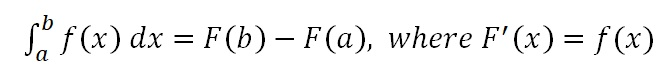WHY IS THIS USEFUL?

Well, suppose you want to find the area under some graph. This theorem gives you a procedure you can follow:

Step 1: Find an antiderivative.

Step 2. See how much the antiderivative changed by over the interval.

For example, suppose we want to know the area under the graph of f(x)=x2 between x=1 and x = 2, as illustrated below.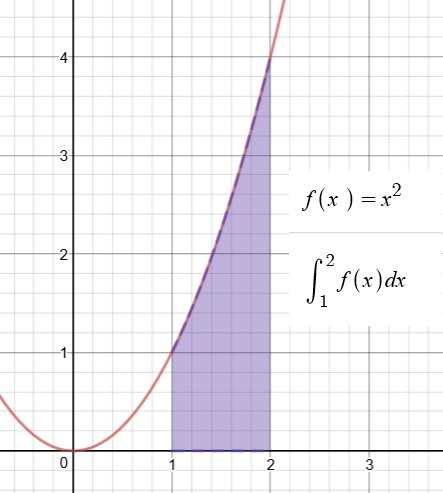Let’s see.  Can we find an antiderivative?  Oh, yes, this one we did already.

F(x)=(1/3)x3 is an antiderivative of  f(x)=x2.

So now we just have to see how much F(x) has changed over the interval from x = 1 to x = 3.

F(3) = (1/3)(2)3 = 8/3

F(1) = (1/3)(1)3 = 1/3

F(3) – F(1) = 7/3

But what if I can’t find the antiderivative?

Well, that could be a problem.  That’s why you will spend so much time developing this skill in AP Calculus.  But I will reassure you again: in AP Physics, the antiderivatives you need will be manageable.  And there’s always Wolfram Alpha

I still don’t see why this is so important?  Are there lots of areas to be found in AP Physics?

Hmm.. No and yes.  See next post…

# A Magical Theorem, Part I: Antiderivatives and Areas

If you have been working your way through these Summer Reading posts, then at this point, you know how to find “derivatives” for a nice collection of reasonable functions.  Nothing too crazy or exotic — just the kinds of functions that you will meet early in AP Physics C.  And you also know why we find derivatives:  derivatives are slope-finding formulas.

For example:

Velocity is the rate of change of position with respect to time.

Acceleration is the rate of change of velocity with respect to time.

Force is the rate of change of momentum with respect to time.

Current is the rate of change of electric charge with respect to time.

For example, suppose you are given that an object moves so that it’s velocity as a function of time is:

v(t) = t2

You can easily find the acceleration as function of time by taking the derivative:

a(t) = v’(t) = 2t

So there are good, useful reasons for learning how to find derivatives. (In fact, let me remind you that to “optimize” a function, you usually begin by finding the derivative and then setting it equal to zero.)

CAN WE PLAY THE GAME IN REVERSE?

If I give you a function, can you find an “antiderivative”?

For example, let’s say:  f(x)=x2

Can we find another function that has f(x)=x2 as its derivative? If we know the basics, finding this particular antiderivative is not that hard:

F(x)=(1/3)x3

But you don’t have to take my word — go ahead an take the derivative!  You will see for yourself that the derivative of F(x)=(1/3)x3 is indeed f(x)=x2.

So  F(x)=(1/3)x3 is an antiderivative of  f(x)=x2.

But it is not the only one!  Try taking the derivative of any of these:

F(x)=(1/3)x3 + 5

F(x)=(1/3)x3 – 99

F(x)=(1/3)x3 + C where C is a constant

So if you can find one antiderivative, you can find a whole family of them.  Still, most times, you only need to find one.

[Caution: your math teacher will likely take off a point or two if you leave out the “…+ c” when finding an antiderivative.]

IS IT ALWAYS THIS EASY?

Well, no.  Some of them are easy.  But others…not so much.  You will spend a substantial amount of time in math class building a collection methods for finding antiderivatives for an increasing variety of functions.  And some of these methods are quite subtle.  For now, I want to make two points:

In our AP Physics class, when the antiderivatives are hard to find, you can have a little help: your TI89s or N-Spires know how to do this.  So does Wolfram Alpha.

Nobody would be willing to invest so much effort in finding antiderivatives unless there was something really useful about them!

A NEW NOTATION

If we are going to be using antiderivatives, we should have symbols for writing them.  To indicate that F(x) is the antiderivative of f(x), we write:This is just another way of saying that F'(x) =  f(x).  And, in addition to calling F(x)  an antiderivative, we also sometimes call it the “indefinite integral of f(x)”.

BUT WAIT…

On the other hand, there is another symbol that looks almost the same but means something completely different: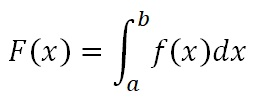This symbol is called the “definite integral” (as opposed to “indefinite”).  It is not a function at all! Just adding values at the top and bottom of that strange elongated s-shape gives the symbol a whole new meaning:

The definite integral is the area of the region bounded by the function, the x-axis and the two “limits of integration”: the vertical lines defined by x=a and x=b.

For example, here is a definite integral: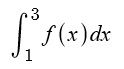And here is the area that definite integral is referring to: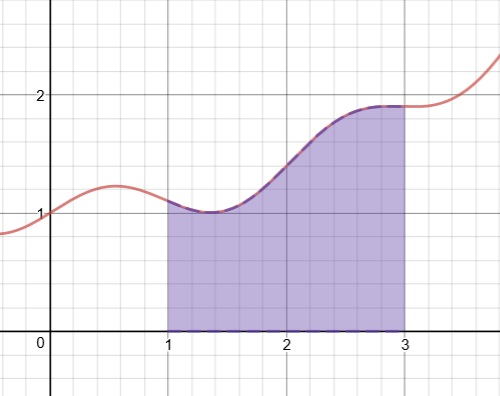So now we have two very different ideas that have been given very similar symbols and names. It would be easy to get these two things confused…

There is the INDEFINITE INTEGRAL:This is a FUNCTION which has f(x) as its derivative.

And then there is the DEFINITE INTEGRAL:This is an AREA — a numeric value.

And now the big question:

Why do mathematicians use these similar names and symbols for these two very different ideas?  Are they secretly related in some FUNDAMENTAL* way?

That is the topic of the next post.

# Enough Calculus to Get Started

An intro for AP Physics C

AP Physics C is a calculus-based course.  At my school, calculus is a co-requisite.  Some of the entering students have already had one quarter of calculus but others will just be starting their first calculus class in September as they start our physics class as well.   I have put together a collection of posts to give everyone an introduction so that we can use calculus from day one.  These posts are their summer reading assignment for AP Physics C.

AP Physics-C is a college-level introduction to Mechanics and E&M (Electricity and Magnetism).  As you will see, many of the ideas of physics are expressed in the language of calculus.  This may sound intimidating but the use of calculus actually makes things easier.  After all, much of calculus was invented for the specific purpose of doing physics.

To help you to a smoother start in physics, I have prepared a series of blog posts that I would like you to read over the summer.  For some of you, most of this will be a review – but definitely not all of it!  But for others, this will all be new. Just take your time, read slowly, and feel free to ask lots of questions — you can save them for September or email me as you think of them!

 POST TOPIC 1 Hairy Questions Slope and Rate of Change 2 Even Hairier Acceleration 3 Velocity NOW! Instantaneous Rate of Change 4 Slope-Finding Functions What a derivative formula tells us 5 Derivatives, Part I: The Building Blocks Derivatives of common functions 6 Derivatives, Part II: Combining Functions Sums, differences, constant product 7 Simple Version of the Chain Rule Composition of Functions 8 Practice Set #1 9 Making the Best of Things Using derivatives for optimization 10 Exponential Decay – Part 1 1st Order Differential Equations 11 Exponential Decay – Part 2 Understanding time constants 12 Practice Set #2 (Will be emailed to you directly)

Students who have successfully completed the two practice sets will have an easy time of it on our first test of the year.  And I believe that you will find that the information will also help you in your calculus class.

One other note:  we will be talking a lot about rotational physics this year.  It would be helpful to have a clear understanding of radian measure.  If you feel at all shaky, you should review these two posts:  Angle Measurement for Pizza Crust Lovers and That Radian Feeling.

If you have any questions about any of these posts, please feel free to email me.  I hope you have a relaxing summer and that you are looking forward to another year of interesting physics.

— Mr. K

# “A positive times a negative” and other mysteries of arithmetic

“Is that the kind of thing you just have to memorize?”

That’s a question that should make a math teacher nervous.  There are certainly procedures and formulas that are occasionally useful to memorize.  But not “just” memorize!  If we don’t aim for a deeper understanding, then we have lost sight of why we are doing any of this.  In fact, if we never even try to have our students understand some particular piece of mathematics, then that piece is a good candidate to drop from the curriculum. (I’m looking at you, Descartes Rule of Signs).

On the other hand, we do want our students to be able to do a lot of things!  And the reasons behind some of them are sometimes more subtle or more advanced than what students need to hear at the moment they are first learning them.  So it can be a bit of a balancing act — finding the right degree of explanation and rigor at every level.

One somewhat disheartening thing I see at the high school level is that my students don’t seem to expect to understand the rules.  They have been graded on their ability to replicate a startling number of procedures.  That these things can be understood seems almost beside the point to them.  I wish we taught a whole lot more about a whole lot less…

So what has me started on this rant on a lovely snow day in the new year?  Well, while not grading a pile of papers, I came across a twitter discussion of this question:

Why is a negative times a negative a positive?

As you ponder this issue, you see that it is related to:

Why is a positive times a negative a negative?

I would like to offer an answer that requires no algebra — so it can be used with the students who are first learning these rules.

GUIDING IDEA

Often in math, we start with an idea that has one interpretation and then we extend it into new areas that cause us to expand or even give up the original interpretation. But we try to extend the idea in ways that continue the rules and patterns that we had before.

So for example, when you first learned to multiply, say 5 × 4 = ?

You can think of that as 5 sets of 4.  (Or 4 sets of 5!)

You can make rows of dots:

•    •    •    •

•    •    •    •

•    •    •    •

•    •    •    •

•    •    •    •

But now someone asks you to multiply a positive times a negative: 5 × -4 = ?

I don’t know how to make 5 rows with -4 dots in a row.  Or -4 rows with 5 dots in each.  So to answer this question, I am going to have to extend what it means to multiply.  But I want to keep patterns intact.  What kind of patterns? Well, here’s one:

5 × 4 = 20

5 × 3 = 15

5 × 2 = 10

5 × 1 = 5

5 × 0 = 0

Hmm.  When you are multiplying 5 by successively smaller whole numbers, your answer decreases by 5 each time.  What if we want that pattern to continue?

5 × -1 = -5

5 × -2 = -10

5 × -3 = -15

5 × -4 = -20  Aha!  That was the question we started with.  We just found a way to multiply a positive by a negative.

[If all you care about is getting the answer, then you memorize the procedure: to multiply a positive by a negative, just multiply the “regular” way and then make your answer negative.  But without some understanding, it feels so hollow.]

But wait!  There’s more…

Now someone asks you to multiply, say:  -4 × -5 = ?

I still don’t know how to draw -5 rows each with -4 dots.  But I can look for patterns!

We have learned how to multiply positives and negatives. So for example…

4 × -5 = -20

3 × -5 = -15

2 × -5 = -10

1 × -5 = -5

0 × -5 = 0

Any pattern?  Hmmm.  When you multiply -5 by successively decreasing whole numbers, the products INCREASE by 5 each time…and if we continue the pattern:

-1 × -5 = +5

-2 × -5 = +10

-3 × -5 = +15

-4 × -5 = +20  Aha (again)!  We have our answer — not because we “just” memorized that a negative times a negative is a positive, but because that is the way it has to be for our pattern to be extended.

The idea of developing new rules in a way that maintains old patterns will certainly be useful again. In fact, I just remembered another example of this.  I once gave a guest lesson to a 5th grade class that wanted to know why a number raised to the power of zero is 1.  I came up with a kind of lengthy explanation involving the laws of exponents…I don’t know how many hearts and minds I won that day.  Then I went home and thought about it a little more.  I came back the following day with a much shorter lesson:

2^5 = 32

2^4 = 16

2^3 = 8

2^2 = 4

2^1 = 2

What happens to the answer each time you lower the exponent by 1?  Keep that pattern going…

# That Trigonometry Diagram Again

Since I started this blog, one recurring goal has been to explore ways to visualize mathematical ideas.  In that spirit,  I spent a few posts talking about this diagram: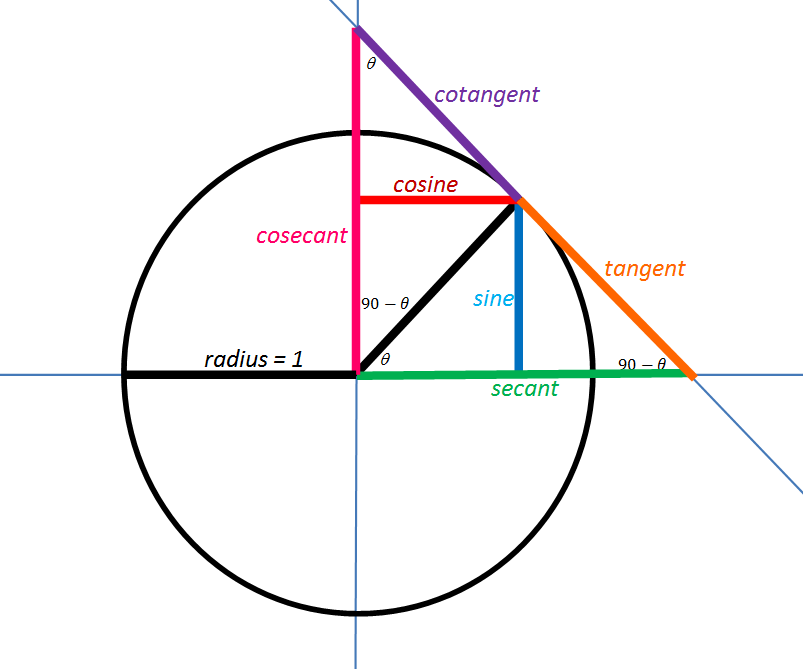These three posts discuss the development of this diagram:

Also Better With Pictures — The Trigonometry Ratios

Picturing the Trigonometric Ratios: Step-by-step

Trig Pictures, Part III

Whenever I show this diagram to students, they seem actually excited to discover the meaning and reasons behind so much trigonometry that they had previously just memorized.  It makes me believe that it would be helpful if this diagram was displayed in every room where trigonometry is introduced.  And now it can be!  I tidied up the graphics and made it into a poster.For your notebook (8.5″ x 11″)  Trig Diagram Notebook-sized

For your wall (22″ x 28″ ) Trig Diagram Poster-Sized

COMING SOON: T-SHIRTS!!  (Maybe)

UPDATE 11/1/2017 My obsession continues…now you can see an animated version.  I made this in Desmos.  You can see it here:

https://www.desmos.com/calculator/mvj7yljovi

Now, how do I get THAT on a t-shirt?

# ALTERNATIVE SOLUTIONS: TEST #8

For more than half of the problems on this test, there is another way to solve the problem, a way that is sneakier, more creative, a little subversive.  It’s good to have more than one way to attack a problem, so read on and learn to play.

DITW = Do it their way

Trial and Error = pick an answer, play with it, see if it fits what they said in the problem.

Back Door = make up numbers for the variables in the problem, work out an answer that is based on the numbers you made up, then put those made up numbers back into the answer choices, ruling out any that don’t produce a matching answer.

More detailed explanations available in my book

PRACTICE TEST #8, SECTION 3: NO CALCULATOR

1. As algebra goes, this is not that bad. But you can also just try the answers. Lazy kids try b first  but no luck. Then they try d and their laziness is rewarded.
1. Well, you should REALLY know how to find a linear equation from a graph. So I guess I am saying DITW. But you can also pick a point on the graph,  say (1,2)  and then see which equation works. If you are unlucky and two answers work (as would happen if you chose m=2, d=4) then pick another point and try again.
1. If you can do the algebra to solve for P, go ahead. But this is also a classic back door problem.  Make up #s that fit, get your answer and then plug your #s into the answer choices.
1. DITW
1. And another back door!  Draw a rectangle, pick a value for w, make the length 6 more than that, find the perimeter and then stick your w number into each answer.
1. DITW
1. Why do algebra?  Try the numbers! 5 works,  -1 does not.  No need to check 0 (but it doesn’t work either).
1. And yet another back door! Try x = 4, see what you get.  Then plug x=4 into each answer.
1. You do have to know that this is a circle centered at (6,-5).  Draw the picture, fill in the center and the endpoint that they gave you: P(10,-5).  Then look at the answers and plot them on your graph.  There will only be one that could be anywhere close to the other end of that diameter.
1. The SAT LOVES this kind of problem.  The official solution shows the algebra method.  They also discuss a slick think-around.  But they forget to mention that you can also use trial and error. For example, say there were 20 2-person tents (B). That takes care of 40 people.  The other 40 of the 60 tents would be 4-person and would hold 160.  That adds up to 200 people, but we need 202.  I bet choice C will work. Try it!
1. If I plug in x=-2, the graph tells me to expect an output around almost -20…try the answer choices.
1. Make up an a and a b that fit: for example, a=1, b=4 — in other words, this is yet another back door problem!
1. While I still think you should know how to write a linear function, note that the right answer has to give an output of 1.9 when the input is 13. So check the answers to see which one does what it’s supposed to.
1. DITW
1. DITW
1. Before doing algebra, a lazy kid checks 1,2 3 and maybe even -1, -2 and -3. Oh, look: 3 works
1. DITW
1. DITW (but they say it funny: just add the equations, lined up as they are.  You get 4y=6, so y=1.5
1. They LOVE this question too: y=ax+b and a is the rate of change, b is the initial value.  If you know that, this is kind of easy to be problem 19.
1. DITW

PRACTICE TEST #8, SECTION 4: WITH CALCULATOR

1. Back door  say c=5. That’s \$10. Now put c=5 into the answers…
1. DITW
1. This is a classic ratio problem: set up thing1/ thing 2 = other thing 1/other thing 2.

4. While you COULD plug x=19 into the equation they give you, you could also estimate directly from the graph.  You have to extend the line a little bit but the answer is clearly a little more than 50 and clearly less than 55.

1. DITW
1. Since you don’t actually have to solve the system, it’s better to DITW.
1. Y=mx+b and the slope is 3.
1. If you see that you can multiply both sides by x+1 to get (x+1)^2, then you take the square root of both sides. That’s their way.  You could also do trial and error.  Or, since this is the calculator section, if you have a ti89 or an nspire, you can let the calculator do the algebra for you.
1. You have a calculator!  They give you a formula.  And they give you answers! Try them one at a time.  When you get 473, stop.
1. An odd and interesting question.  But DITW.
1. Division, or just trial and error…
1. DITW
1. This will be an easy back door play as long as you know what a negative exponent means and what ½ as an exponent means. When it says a ^(-1/2) that means “one over the square root of a”.  So if you make a=25, x will be 1/5.  Now go to the answer choices, plug in 1/5 and see which comes out as 25.
1. It will be undefined when the denominator = 0. But you can skip the algebra and just try the answer choices.
1. DITW
1. DITW  but again I have to complain about the wording.  They say “what proportion” when they should really say “what fraction”.  I know I am nitpicking, but still…
1. If you understand their way that’s fine. But many people find it easier to understand if they list out all the data:

18,18,18,18,18,18, 19, 19, 19, 19, 19, 20,20,20,20,20, 21,21,22,23,30

Notice how much easier it is now to find mean, median and mode.

1. DITW
1. DITW
1. DITW
1. Yet another question testing your ability to interpret y=mx+b. The m is the slope which is also the rate of change.
1. DITW
1. I am sure that many students who think they are too advanced to bother making up numbers will actually get this wrong (by putting b). Say t=3. Calculate 1800(1.02)^3.

In 3 years, there are  q=12 quarters.  Put q=12 into each answer choice.  You will see that it is a and not b that’s the answer you were looking for.

24. Well, DITW…but also: you get this wrong by assuming things that the problem never said.  In the math section, as in the reading section, that will burn you every time.

1. Yes, you could find the slope and intercept and then write the equation…but here’s something helpful to notice: when you plug t = 10 into the right answer, you better get 846 back as your answer.  Start calculating!
1. DITW
1. DITW and note that this is a righteously challenging question, as it should be for its location in the section.
1. You do not need to know how to calculate standard deviations. You just need an intuitive feeling that if the data is clustered around the mean, the std. deviation is low and if it is spread out, the std deviation is higher. Then, you can just eyeball this one.
1. First of all, eliminate c and d — they are exponential but the problem says “constant rate” so you know we need linear graphs. And after 20 minutes, 30% of the paper is gone so 70% remains or 3500 sheets. So of the two answers, pick the one that comes out to 3500 when you plug in m = 20.
1. DITW and it’s a good problem!
1. Remember: early grid-in should be easy.  Don’t be psyched out by the chemistry.  You have 51 thingies.  They each contain two smaller thingies of one kind and one of a second kind.  So overall, you have 102 of the first kind and 51 of the second kind.
1. Stick in x=1. Algebra or trial and error to find what works.
1. If you multiply both sides of an equation by a constant, it’s an equivalent equation with the same solutions and the same graph!  It’s easy to see that the constant they multiplied by this time was 3. So 10 x 3 = 30.
1. DITW
1. Yes, you can set the two equations equal and do the algebra.  Or you can let your ti89 do it.  Or with any graphing calculator, you can graph both equations and then trace along until you find the intersection.  But my laziest students draw the g function’s graph by hand! You know it starts at (0,10) and it has a slope of -1. So go to (0,10). Then,go down 1, over 1, down 1, over 1 (watching the scale of the graph). In two steps, you will land on the first intersection point.
1. This is exactly the kind of question that rewards thorough SAT prep. It looks very hard.  But then you notice that the angles they ask about are complementary. And you know the rule for complementary angles: sine of one = cosine of the other. So they subtract to get zero.  If you take 5 practice tests, you will see this concept tested 3 times.
1. Just read the chart and be careful: for the first sample, you multiply by 10, for the second you multiply by 8 and then you subtract the two results. (Which is, to be fair, DITW)
1. And this is DITW as well.

OK, I count more than 30 out of the 58 that have at least one alternative solution!

# Exponential Decay and Time Constants

The other day, a colleague showed me a diagram about current vs. time in an RC circuit. The diagram helped me realize that there is another way to think about time constants and exponential decay, a way I had completely overlooked.  I had all the right pieces floating around but I had never put them together.

WHAT’S A TIME CONSTANT?

Here are three graphs showing a quantity that is decaying exponentially toward zero: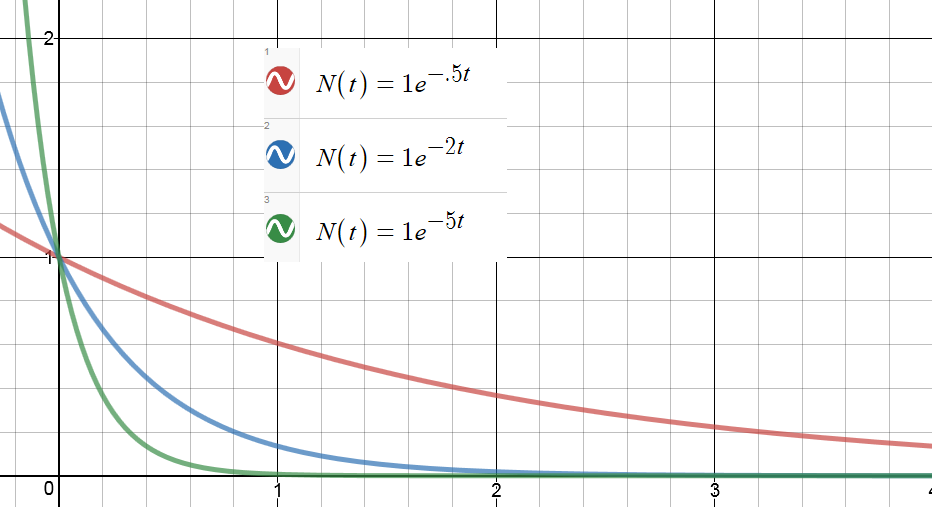Each of these is a graph of a function: N(t) = Noe-kt

The only difference is the value of the constant, k.  Higher values of k lead, in a sense, to faster decay.

To help emphasize this, we can define a constant: τ = 1/k

Then we can  re-write the function this way: N(t) = Noe-t/τ

We call τ the “time constant” for this decay.  It has the units of time.  And it gives us an intuitive feeling for how fast a function is decaying.

For every time constant that passes, our decaying quantity gets reduced by another factor of e.

So after one time constant has passed, the function’s value is No/e.  After two time constants, it’s No/e2.  After three, No/e3 …and so on.

But there is another way to think about the time constant.

The time constant tells us how long it would take to reach the asymptote at the current rate of change.

Maybe this should have been obvious, but I never thought about that way until just a few days ago. Here’s the diagram that got me started: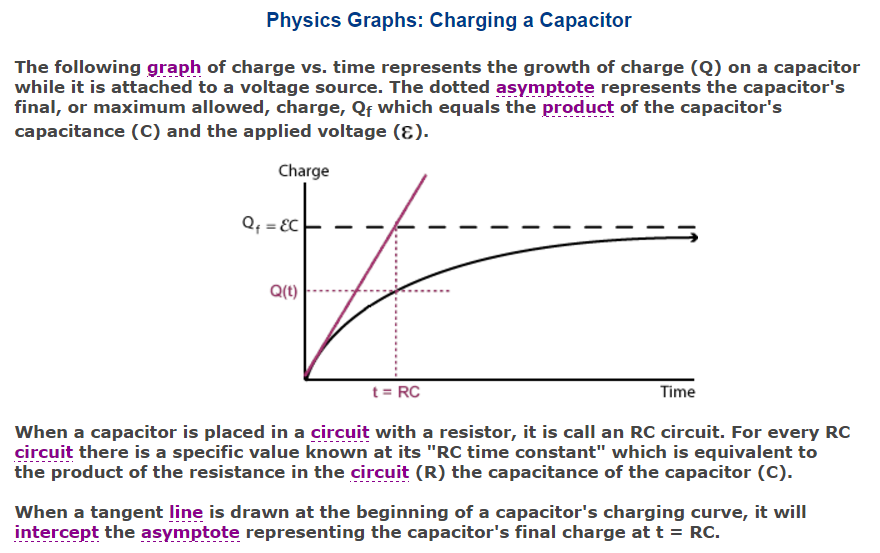The claim here is made in the context of a charging capacitor, but it is actually true for any function that is approaching an asymptote exponentially. Here is an animation I made in Desmos to illustrate the point:

In the still picture above, you can see the tangent line at t=0.  The dotted green segment on the t-axis represents the time it would take for the tangent line to reach the asymptote.  When you run the video, you will see the function decrease and the tangent line get shallower.  But that green segment stays the same length.

(If you want to play with the Desmos file, you can get it here🙂

WAIT – WHAT’S A TIME CONSTANT NOW?

A quantity that decays exponentially approaches its final value asymptotically. And as it decays, its rate of decay decreases as well.  (That’s the defining characteristic of exponential decay: the rate of decay toward the final value is proportional to the current distance from that value.  In fact, we can write that as a differential equation. I went on and on about that here.)

But now suppose that at some moment, the decay rate were to become constant. Instead of taking forever, the function would now be able to reach its asymptote in a finite amount of time. Not in just any old amount of time, but in fact in an amount that equals the “time constant” of the decay. It doesn’t matter what moment you pick.  From any starting point during exponential decay, if the rate were to stay constant (which it doesn’t, but still…) the time to reach the asymptote would always comes out to τ = 1/k.

Or to say the same thing geometrically: the tangent line from any point on the curve will intercept the asymptote after one time constant has elapsed. That’s what the animation is trying to emphasize.  I chose a k value of .2 so my time constant was 1/.2 = 5 seconds.  If you open the Desmos file, you can change the constants to see what changes and what doesn’t.

SO WHY DID THIS SURPRISE ME?

I wasn’t making the connection between the exponential decay and the differential equation lurking in the background (even though I teach that connection and have blogged about it).

That the function decreases with a rate of change that is proportional to the value of the function is actually a first-order differential equation.  The exponential decay is its solution. (Again, gory detail here.)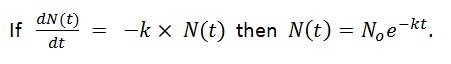And if slope is rise over run, then run is rise over slope!  When the function is starting from a higher value, the tangent has a slope that is PROPORTIONALLY higher!  So that proportionally higher slope will get you back to the asymptote in the same amount of time every time.

To find how much time we are talking about, divide the “rise” (or in this case, a “fall”) by the slope: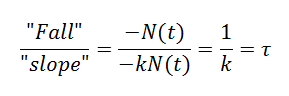I’ll leave a more rigorous, math-y proof as an exercise for those of you in your first calculus class.